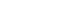# Loose Leaf Engineering Circuit Analysis Chapter 2, Problem 6P

Question: Determine the power being absorbed by the circuit element in Fig.2.14a.

FIGURE 2.14

Solution:

The following is the given circuit element:

Figure 1

Calculate the power absorbed by the element.

Therefore, the value of power absorbed is.